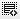What is the difference between Depreciation and Amortization?0
Explain in brief0

Amortization usually refers to spreading an intangible asset's cost over that asset's useful life.Depreciation, on the other hand, refers to prorating a tangible asset's cost over that asset's life0

Depreciation is a method in which capital expenditure is expensed. It is a way of expensing tangible(hard objects). An example to be considered is the life cycle of an asset(oven), and how it decreases over a period of time.
Ammortization is long term investment expensed over a period of time. It is a way of expensing intangible objects. Research and Development usually uses Ammortization.0

Depreciation is the method of expensing the capital expenditure in the balance sheet into the income statement ie on Tangible Asset while Armortisation is a method of expesing an intangible asset over a period of time.0

Depreciation is the scheduled charging to expense of a tangible asset over its useful life. Amortization is the scheduled charging to expense of an intangible asset over its useful life. Thus, the key difference between amortization and depreciation is that one relates to intangible assets, and the other to tangible assets.
Another difference between the two concepts is that amortization is almost always conducted on a straight-line basis, so that the same amount of amortization is charged to expense in every reporting period. Conversely, it is more common for depreciation expense to be recognized on an accelerated basis, so that more depreciation is recognized during earlier reporting periods than later reporting periods.

Yet another difference between amortization and depreciation is that the calculation of amortization does not usually incorporate any salvage value, since an intangible asset is not typically considered to have any resale value once its useful life has expired. Conversely, a tangible asset may have some salvage value, so this amount is more likely to be included in a depreciation calculation.0

Depreciation is a way of expensing the cost of a tangible asset over a period of time whereas
Amortization is a way of expensing the cost of an intangible asset over a period of time0

depreciation is charged only on fixed assets which is visible and tangible.,
whereas
amortization is a charge applicable for intangible assets like goodwill, patents , trademarks etc0

Depreciation is the systematic and periodic allocation of the historical cost or revalued amount less estimated residual value of a depreciable asset over its estimated useful life.

Amortization means spreading a payment over a period of time . Example: Insurance is paid once, but the amount is spread every month. If the premium is \$12000, it will be \$1000 monthly.0

Amortization usually refers to spreading an intangible asset's cost over that asset's useful life. For example, a patent on a piece of medical equipment usually has a life of 17 years.

Depreciation on the other hand, refers to prorating a tangible asset's cost over that asset's life. For example, an office building can be used for a number of years before it becomes run down and is sold.

Click on thisicon to add code snippet.